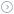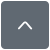### 0802LCD 4位显示程序 PIC单片机C语言程序

|

#include

#include

#define uchar unsigned char

#define uint unsigned int

#pragma config FOSC = XT        // Oscillator Selection bits (XT oscillator)

#pragma config WDTE = OFF       // Watchdog Timer Enable bit (WDT disabled)

#pragma config PWRTE = ON       // Power-up Timer Enable bit (PWRT enabled)

#pragma config BOREN = OFF      // Brown-out Reset Enable bit (BOR disabled)

#pragma config LVP = ON        // Low-Voltage (Single-Supply) In-Circuit Serial Programming Enable bit (RB3 is digital I/O, HV on MCLR must be used for programming)

#pragma config CPD = OFF        // Data EEPROM Memory Code Protection bit (Data EEPROM code protection off)

#pragma config WRT = OFF        // Flash Program Memory Write Enable bits (Write protection off; all program memory may be written to by EECON control)

#pragma config CP = OFF         // Flash Program Memory Code Protection bit (Code protection off)

//char tab[]="0123456789";

#define uchar unsigned char

#define uint unsigned int

void LCD_write_cmd(uchar command);

void LCD_write_command(uchar command);

void LCD_en_write(void);

void LCD_set_xy( unsigned char x, unsigned char y );

void LCD_write_string(unsigned char X,unsigned char Y,unsigned char *s);

void LCD_write_data(unsigned char data);

void delay_nus(unsigned int n);

void delay_nms(unsigned int n);

#define RS RC2

#define EN RC3

#define DB7 RC7

#define DB6 RC6

#define DB5 RC5

#define DB4 RC4

#define LCD_DATA_PORT PORTC

#define LCD_DATA_TRIS TRISC

/*------------------------------------------------------------------------------

函数说明

------------------------------------------------------------------------------*/

void LCD_init()

{

TRISD=0x00; //数据口方向为输出

PORTD=0x00;

TRISC=0x00; //数据口方向为输出

PORTC=0x00;//设置EN、RS/数据为输出

LCD_write_cmd(0x30);//4位的指令

delay_nms(5);

LCD_write_cmd(0x30);

delay_nus(200);

LCD_write_cmd(0x30);

delay_nms(1);

LCD_write_cmd(0x20);

LCD_write_cmd(0x20); //4位显示

LCD_write_cmd(0x80);

LCD_write_cmd(0x00); //显示开 游标、闪烁不显示

LCD_write_cmd(0x80);

LCD_write_cmd(0x00); //清屏

LCD_write_cmd(0x01);

LCD_write_cmd(0x00); //两行 5*7

LCD_write_cmd(0xc0);

}

void LCD_write_cmd(uchar command) //写指令

delay_nus(10);

RS=0;

EN=0;//使能清零

LCD_DATA_PORT&=0x0f; //清除端口

delay_nus(2);

EN=1;

LCD_DATA_PORT |= (command & 0xf0);//高4位不用改

delay_nus(2);

EN=0;

}

void LCD_write_command(uchar command) //写指令

LCD_write_cmd(command);

LCD_write_cmd(command<<4);

}

void LCD_write_data(unsigned char data) //写数据

delay_nus(10);

RS=1;

EN=0;//使能清零

LCD_DATA_PORT&=0x0f;

EN=1;

LCD_DATA_PORT |= (data & 0xf0);//高4位不用改

delay_nus(2);

EN=0;

delay_nus(2);

LCD_DATA_PORT&=0x0F; //清低四位

EN=1;

LCD_DATA_PORT |= ((data << 4) & 0xf0);//发送低4位

delay_nus(2);

EN=0;

}

void LCD_write_string(unsigned char X,unsigned char Y,unsigned char *s) //列x=0~15,行y=0,1

LCD_set_xy( X, Y ); //写地址

while (*s) // 写显示字符

{

LCD_write_data( *s );

s ++;

}

void LCD_set_xy( unsigned char x, unsigned char y )  //写地址函数

if (y == 0) address = 0x80 + x;

else        address = 0xc0 + x;

void delay_nms(unsigned int n) //N ms延时函数

uint a,b;

for(a=n;a>0;a--)

for(b=80;b>0;b--);

void delay_nus(unsigned int n) //N us延时函数

unsigned int i;

for (i=0;i

}

void main()

{

LCD_init();

//    LCD_write_command(0x0d); //光标开

while(1)

{

RD0=1;

delay_nms(1000);

RD0=0;

delay_nms(1000);

LCD_write_string(0,0,"ceshiLCD");

LCD_write_string(0,1,"hahahaha");

delay_nms(2000);

}

}

『本文转载自网络,版权归原作者所有,如有侵权请联系删除』热门文章 更多footer